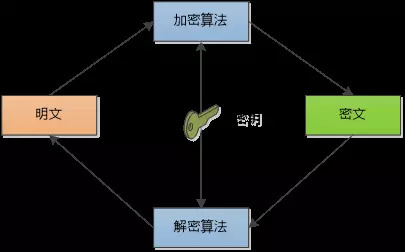# 两个程序的爱情故事（续）“那更不行了， Copy到U盘上更容易泄露，速度还慢！ ”

1. 首先我和她先协定一个质数 p=17以及另外一个数字g=3， 这两个数字是公开的， 黑客拿去也没有问题

2. 我选择一个随机的秘密数字x = 15, 计算a = g15 mod p并发送给她。
a = 315 mod 17 = 6.

3. 她选择一个随机的秘密数字y=13, 计算b = g13 mod p并发送给我。
b = 313 mod 17 = 12.

4. 我拿到她发给我的b = 12 , 计算s = b x mod p ->1215 mod 17 = 10

5. 她拿到我发给她的a = 6, 计算s = a y mod p -> 613 mod 17 = 10

（注：例子来源于wikipedia, 红色表示数字一定要保密， 绿色表示数字可以公开）

“可是为什么会这样呢” 我问道。

“数学家小帅哥说了， 原因很简单，(gｘ mod p)ｙ mod p　和　(gｙ mod p)ｘ mod p　是相等的！ ”

“那黑客不能从公开传输的 p = 17, g = 3, a = 6 , b = 12 推算出s = 10 吗？” 我问道。

“当然不能， 不过前提是需要使用非常大的p , x, y, 这样以来，即使黑客动用地球上所有的计算资源， 也推算不出来。 ”